# 0x01 前端要做的事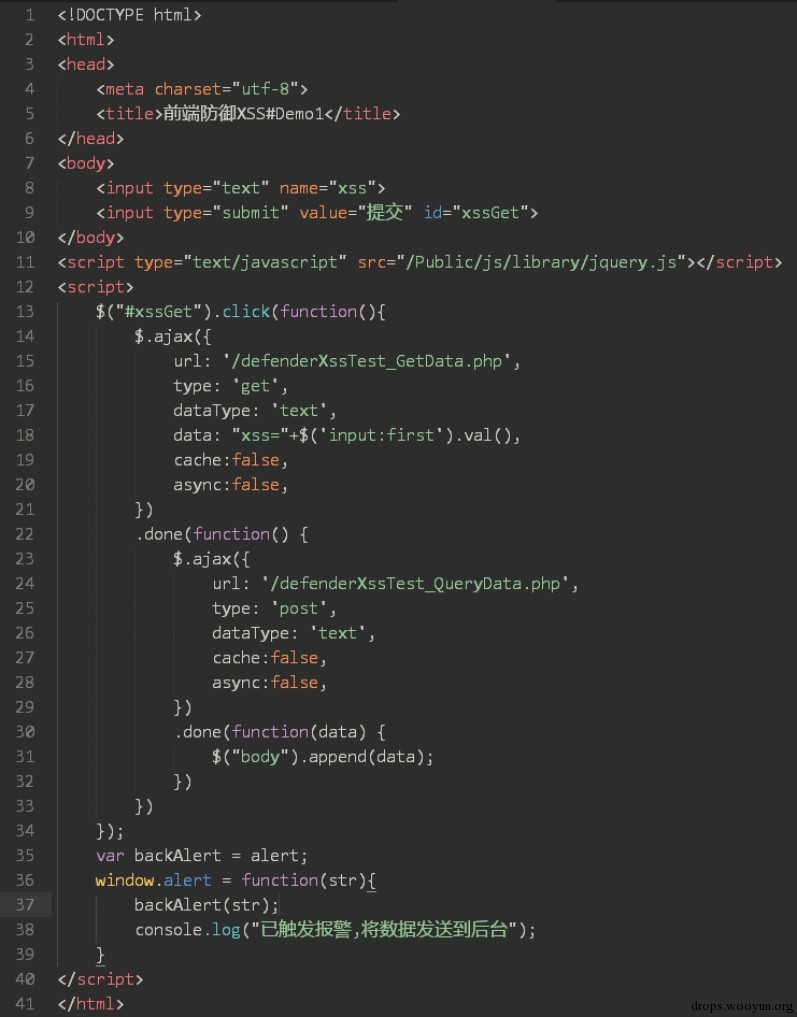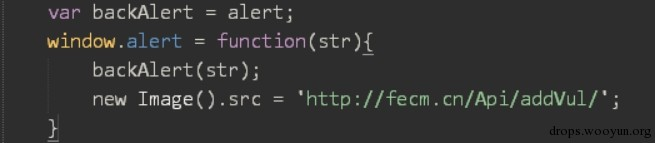# 0x02 数据库要做的事

fecm_user的字段信息如下：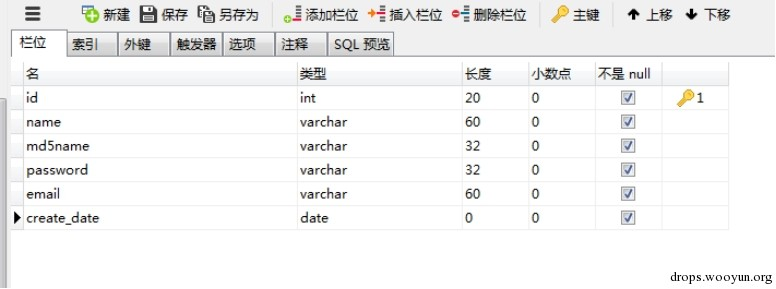• name：管理员账户名
• md5name：3次name值的md5
• email：管理员邮箱
• create_date：管理员创建时间

fecm_bugdata的字段信息如下：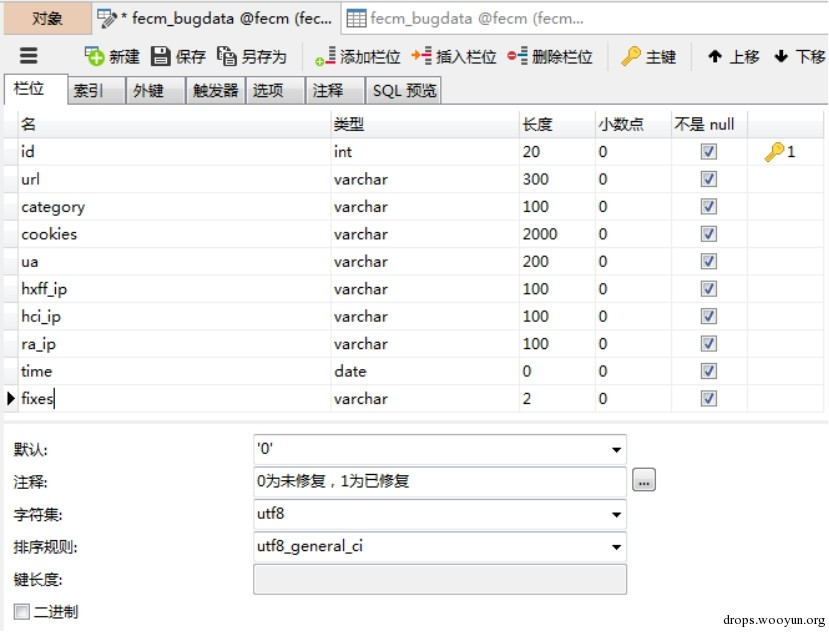• url：漏洞的url地址
• category：漏洞类型
• ua：攻击者的User-Agent
• hxff_ip：攻击者的HTTP_X_FORWARDED_FOR
• hci_ip：攻击者的HTTP_CLIENT_IP
• time：攻击者攻击的时间
• fixes：漏洞是否修复（0为未修复，1为已修复）

# 0x03 后端要做的事

``````#!php
if(I('get.category','','int') == ""){
\$this->ajaxReturn(array(
"typeMsg" =>  "error",
"msgText" =>  "漏洞类型错误",
));
}
switch (I('get.category','','int')) {
case '1':
\$vul['category'] = "触发alret函数";
break;
case '2':
\$vul['category'] = "发现不在白名单里的第三方JavaScript资源";
break;
default:
\$this->ajaxReturn(array(
"typeMsg" =>  "error",
"msgText" =>  "漏洞类型错误",
));
break;
}
if(\$_SERVER['HTTP_X_FORWARDED_FOR'] === null){
\$vul['hxff_ip'] = "攻击者没有通过代理服务器访问";
}else{
\$vul['hxff_ip'] = I('server.HTTP_X_FORWARDED_FOR'); //获取攻击者的HTTP_X_FORWARDED_FOR
}
if(\$_SERVER['HTTP_CLIENT_IP'] === null){
\$vul['hci_ip'] = "攻击者数据包头部没有HTTP_CLIENT_IP";
}else{
\$vul['hci_ip'] = I('server.HTTP_CLIENT_IP');//获取攻击者的HTTP_CLIENT_IP
}
}
\$vul['url']   = I('server.HTTP_REFERER');   //获取攻击者攻击成功的url
\$vul['ua']    = I('server.HTTP_USER_AGENT');    //获取攻击者的User-Agent
\$vul['time']  = date("Y-m-d");  //获取攻击者攻击的时间
\$vul['fixes'] = 0;  //默认为漏洞未修复
\$bugData = M('bugdata');    //连接fecm_bugdata数据库
}
``````

``````#!php
public function index(){
\$reportForm = M('bugdata'); //连接fecm_bugdata数据库
\$dateTimeLabels = [];
\$dateTimeTotal = [];
for(\$i = 0;\$i < 7;\$i++){    //获取近7天的数据
\$time = date("Y-m-d",strtotime(-\$i." day"));
array_unshift(\$dateTimeLabels,\$time);
\$data['time'] = array('like','%'.\$time.'%');
array_unshift(\$dateTimeTotal,\$reportForm->where(\$data)->count());
}
\$reportForm = json_encode(["Labels" => \$dateTimeLabels,"Total" => \$dateTimeTotal]); //转化成json格式
\$this->assign('reportForm',\$reportForm)->assign('total',total());   //交给前端模块
\$this->display();   //前端页面生成
}
``````

``````#!js
var lineChartData = {
labels :eval({\$reportForm})['Labels'],
datasets : [
{
fillColor : "rgba(151,187,205,0.5)",
strokeColor : "rgba(151,187,205,1)",
pointColor : "rgba(151,187,205,1)",
pointStrokeColor : "#fff",
data : eval({\$reportForm})['Total']
}
]
}
var myLine = new Chart(document.getElementById("Statistics").getContext("2d")).Line(lineChartData);
``````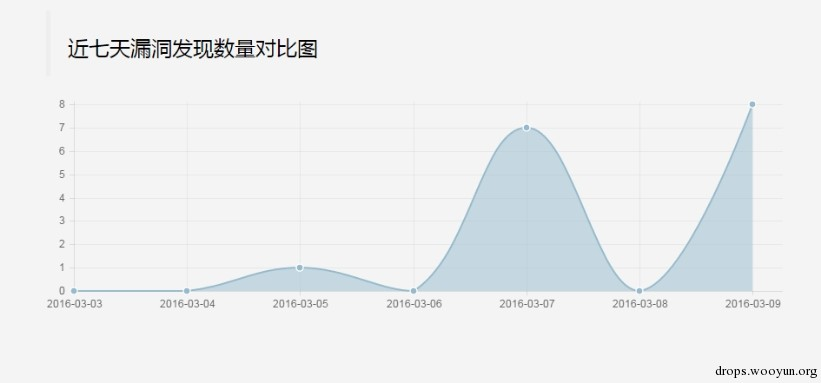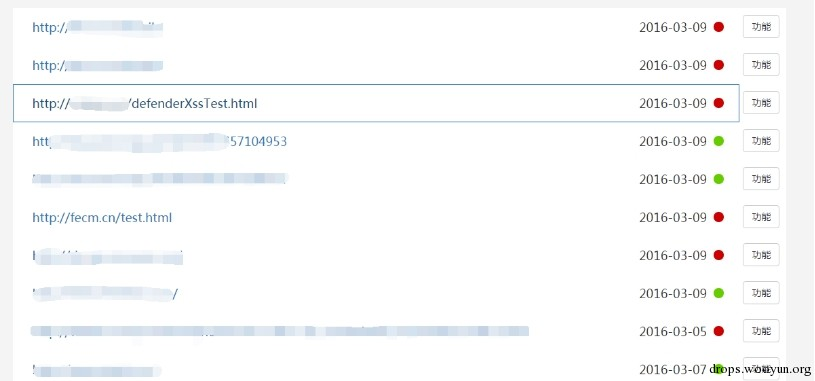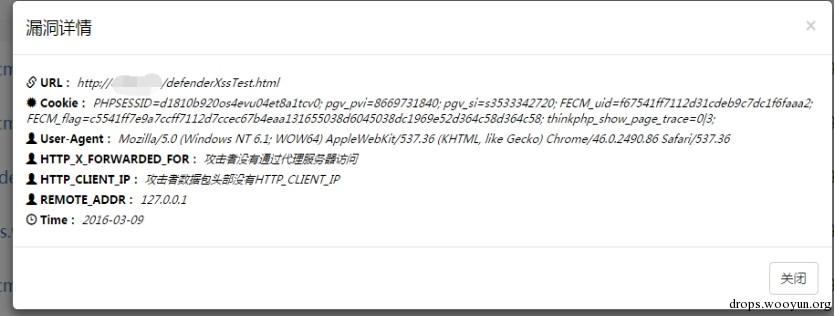# 0x04 让我们实际测试一下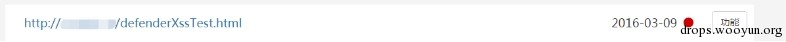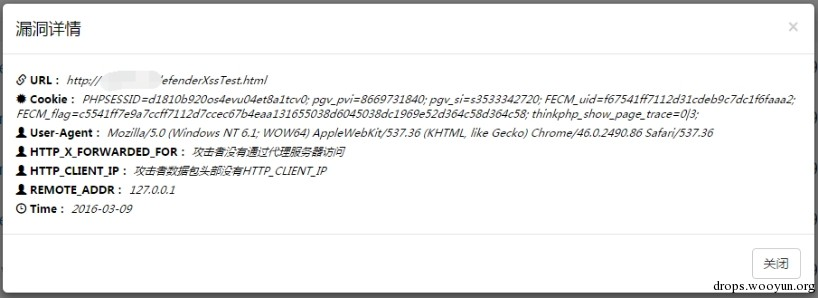# 0x05 检测第三方js资源是否为xss脚本

``````#!js
var a = document.createElement('a');
a.href = tag.src||tag.href||tag.data;
if(a.hostname!=location.hostname){
console.warn(location.hostname+' 发现第三方资源['+tag.localName+']:'+a.href);
}
}
``````

``````#!js
var scriptList = [
location.hostname,
]
``````

``````#!js
var webScript = document.querySelectorAll('script[src]');
``````

``````#!js
for(var i = 0;i < webScript.length;i++){
var a = document.createElement('a');    //建立一个新的a标签，方便取值
a.href = webScript[i].src;  //把script里的src赋值给a标签里的href属性
if(a.hostname != webHost){  //对比，是否为第三方资源
for(var j = 0;j < scriptList.length;j++){
if(a.hostname != scriptList[i]){    //判断当前的第三方资源是否在白名单里
}
}
}
}
``````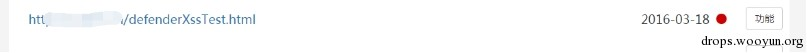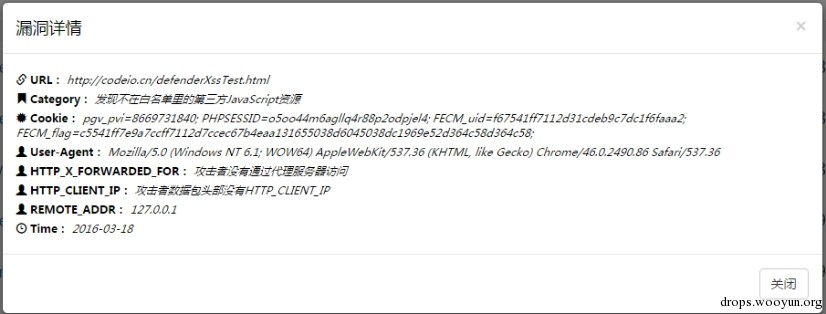# 0x06 结语[微信] 扫描二维码打赏[支付宝] 扫描二维码打赏